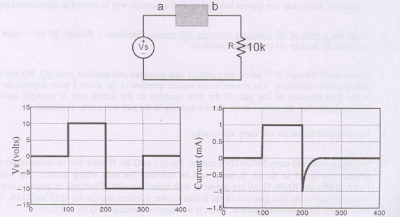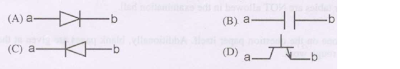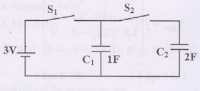## Saturday, November 24, 2012

### Basic Electrical Engineering: Part9 Exam Objective Questions

Basic Electrical Engineering Solved Objective Questions from Competitive Exams
 Consider the following statements:  [IES2010]
Piezoelectric materials serve as
1. A source of ultrasonic waves
2. When electric field is applied, the mechanical dimensions of the substances are not at all altered.
3. Converts electrical energy to mechanical and vice versa
4. Converts thermal energy to electrical energy
Which of the above statements is/are correct?
A. 1 only
B. 2 and 3 only
C. 1 and 3 only
D. 1,2,3 and 4

The current through the 2KΩ resistance in the circuit shown is [GATE2009]

A. 0 mA
B. 1 mA
C. 2 mA
D. 6 mA

 Out of the following plant categories
1.Nuclear
2.Run-of-river
3.Pump storage
4.Diesel
the base load power plants are [GATE2009]
A.1 and 2
B. 2 and 3
C.1,2 and 4
D.1, 3,and 4

 The following circuit has a source voltage Vs as shown in the graph. The current through the circuit is also shownThe element connected between a and b could be [GATE 2009]Each Question Carries Two Marks Each
 The following circuit has R= 10kΩ , C=10μF. The input voltage is a sinusoid at 50Hz with an rms value of 10V. Under ideal conditions, the current is from the source is [GATE 2009]A.  10π mA leading by 90°
B.  20π mA leading by 90°
C.  10π mA leading by 90°
D.  10πmA lagging by 90°

 In the figure shown, all elements used are ideal. For time t<0, S1 remained closed and S2 open. At t=0, S1 is opened and S2 is closed. If the voltage Vc2 across the capacitor C2 at t=0 is zero, the voltage across the capacitor combination at t=0+ will be [GATE 2009]A.  1V
B.  2V
C.  1.5V
D.  3 V

 Transformer and emitter follower can both be used for impedance matching at the output of an audio amplifier. The basic relationship between the input power Pin and output power Pout in both the cases is [GATE 2009]
A.  Pin = Pout for both transformer and emitter follower
B.  Pin > Pout for both transformer and emitter follower
C.  Pin < Pout for transformer and Pin = Pout for emitter follower
D.  Pin = Pout for transformer and Pin < Pout for emitter follower

 The equivalent capacitance of the input loop of the circuit shown is [GATE 2009]A.  2μF
B.  100μF
C.  200μF
D.  4μF

 An UJT exhibits negative resistance region
(a) Before the peak point
(b) Between peak and valley points
(c) After the valley point
(d) Both (a) and (c)
OPTIONS:
1) B only
2) A, C
3) C only
4) D only
5) None of the above options

 In an UJT, maximum value of charging resistance is associated with
(a) Peak point
(b) Valley point
(c) Any point between peak and valley points
(d) After the valley point
OPTIONS:
1) A only
2) A, B
3) C only
4) D only
5) None of the above options

 When an UJT is used for triggering an SCR, the wave shape of the voltage obtained from UJT circuit is
(a) Sine wave
(b) Saw-tooth wave
(c) Tapezoidal wave
(d) Square wave
(e) None of the above

 In a LVDT, the secondary voltages [Gate 1997]
a) Are independent of the core position
b) Vary unequally depending on the core position
c) Vary equally depending on the core position
d) Are always in phase quadrature

 In a microprocessor, the address of the next instruction could be executed, is stored in [Gate 1997]
(a) Stack pointer
(c) Program counter
(d) General purpose register

 If a diode is connected in anti parallel with a SCR, then [Gate 1997]
(a) Both turn off power loss and turn off time decrease
(b) Turn off power loss decreases, but turn off time increases
(c) Turn off power loss increases, but turn off time decreases
(d) The arrangement works as a triac

Solution Hint:
 Redraw the circuit as follows

Here R1R4 = R2R3 = 1
ie, it is a balanced bridge, so I = 0mA

 For t < 0, S1 is closed and S2 is opened so the capacitor C1 will charged upto 3 volt.
VC1(0+ ) = 3 Volt
Now when switch positions are changed, by applying charge conservation
VC2(0+ )  = 3 Volt

 Remember that
For transformer, power will be same in input and ouput side. Only voltage/current will be steped up or down.
ie, Pin = Pout
So options B, C will not be applicable.
For emitter follower,
voltage gain = 1 and current gain >1
Pout = [voltage gain] x [current gain] x [Pin]
So option A is correct

 Apply KVL to the input loop
v1 - i1(1+1)x103 - [1/jωC](i1+49i1) = 0
Rearrange the above equation for v
Devide it to get input impedance [Z =  v1/i1] = 2x103 + [1/jωC/50]
Ceq = C/50 =100μF/50 = 2μF
6:03 AM

#### 1 comment:

1.